# magi-vignette

This vignette provides a brief introduction to our R software package for the MAGI (MAnifold-constrained Gaussian process Inference) method (Yang, Wong, and Kou 2021, PNAS) for dynamic systems. For detailed usage, see our companion article on arXiv at https://arxiv.org/abs/2203.06066 and the R package documentation.

Ordinary differential equations (ODEs) are widely used as models for dynamic systems in application domains, including gene regulation, economics, chemistry, epidemiology and ecology. We focus on the setting where the ODEs are nonlinear and unknown parameters govern their behavior. The problem of interest is to recover the system trajectories and to estimate the parameters from experimental or observational data, where the observations taken from the system may be subject to measurement noise and may only be available at a sparse number of time points. Further, some components in the system may not be observable. MAGI has been shown to provide fast and accurate inference for this parameter estimation problem, including the case when there are unobserved system components.

We provide two example systems in this vignette to illustrate usage of the package. We begin by loading the package.

library("magi")
#> ##  See vignette https://arxiv.org/abs/2203.06066 for detailed usage instructions,
#> ##  and https://github.com/wongswk/magi for additional documentation.
#> ##
#> ##  Please cite MAGI method as:
#> ##  Yang, S., Wong, S.W. and Kou, S.C., 2021.
#> ##  Inference of dynamic systems from noisy and sparse data via manifold-constrained Gaussian processes.
#> ##  Proceedings of the National Academy of Sciences, 118(15), e2020397118.

The core function of the package is MagiSolver, which generates MCMC samples (via Hamiltonian Monte Carlo) of the parameters and trajectories from their posterior distribution. The basic syntax of Magisolver is

MagiSolver(y, odeModel, control = list())

where y is a data matrix, odeModel is a list of ODE functions and inputs, and control provides optional control variables.

## Basic usage example: Fitzhugh-Nagumo equations

The Fitzhugh-Nagumo (FN) equations describe spike potentials and consists of two system components $$X = (V,R)$$, which follow the ODE

$\mathbf{f}(X, \theta, t) = \begin{pmatrix} c(V-\dfrac{V^3}{3}+R) \\ -\dfrac{1}{c}(V-a+bR) \end{pmatrix}$ where $$\theta=(a,b,c)$$ are the parameters of interest. Suppose noisy measurements are taken for $$V$$ and $$R$$ from this system at time points $$t= 0, 0.5, 1, \ldots, 20$$, as given below:

tvec <- seq(0, 20, by = 0.5)
V <- c(-1.16, -0.18, 1.57, 1.99, 1.95, 1.85, 1.49, 1.58, 1.47, 0.96,
0.75, 0.22, -1.34, -1.72, -2.11, -1.56, -1.51, -1.29, -1.22,
-0.36, 1.78, 2.36, 1.78, 1.8, 1.76, 1.4, 1.02, 1.28, 1.21, 0.04,
-1.35, -2.1, -1.9, -1.49, -1.55, -1.35, -0.98, -0.34, 1.9, 1.99, 1.84)
R <- c(0.94, 1.22, 0.89, 0.13, 0.4, 0.04, -0.21, -0.65, -0.31, -0.65,
-0.72, -1.26, -0.56, -0.44, -0.63, 0.21, 1.07, 0.57, 0.85, 1.04,
0.92, 0.47, 0.27, 0.16, -0.41, -0.6, -0.58, -0.54, -0.59, -1.15,
-1.23, -0.37, -0.06, 0.16, 0.43, 0.73, 0.7, 1.37, 1.1, 0.85, 0.23)

MAGI is used to recover the system trajectories and to estimate the parameters, as follows.

First, we create a function fnmodelODE that encodes the ODEs. It takes as input the vector of parameters $$\theta$$ and system states $$X$$ one per column and time $$t$$, and returns an array with the values of the derivatives $$\dot{X}$$ at each input time point:

fnmodelODE <- function(theta, x, tvec) {
V <- x[, 1]
R <- x[, 2]

result <- array(0, c(nrow(x), ncol(x)))
result[, 1] = theta * (V - V^3 / 3.0 + R)
result[, 2] = -1.0/theta * (V - theta + theta * R)

result
}

Second, to facilitate MCMC sampling via Hamiltonian Monte Carlo (HMC), we also supply functions for the gradients of the ODE with respect to the system components $$X$$ and the parameters $$\theta$$. With respect to $$X$$, we have the matrix of gradients as follows,

$\frac{\partial \mathbf{f}(X, {\theta}, t)}{\partial X} = \begin{pmatrix} c(1-V^2) & c \\ -1/c & -b/c \end{pmatrix}$ which we specify by defining the function:

# Gradient with respect to system components X
fnmodelDx <- function(theta, x, tvec) {
resultDx <- array(0, c(nrow(x), ncol(x), ncol(x)))
V = x[, 1]

resultDx[, 1, 1] = theta * (1 - V^2)
resultDx[, 2, 1] = theta

resultDx[, 1, 2] = -1.0 / theta
resultDx[, 2, 2] = -theta / theta

resultDx
}

With respect to $$\theta$$, we have the matrix of gradients as follows,

$\frac{\partial \mathbf{f}(X, {\theta}, t)}{\partial{\theta}} = \begin{pmatrix} 0 & 0 & V-V^3/3 + R \\ 1/c & -R/c & (V - a + bR)/c^2 \end{pmatrix}$ which we specify by defining the function:

# Gradient with respect to parameters theta
fnmodelDtheta <- function(theta, x, tvec) {
resultDtheta <- array(0, c(nrow(x), length(theta), ncol(x)))

V = x[, 1]
R = x[, 2]

resultDtheta[, 3, 1] = V - V^3 / 3.0 + R

resultDtheta[, 1, 2] =  1.0 / theta
resultDtheta[, 2, 2] = -R / theta
resultDtheta[, 3, 2] = 1.0 / (theta^2) * (V - theta + theta * R)

resultDtheta
}

We may wish to verify that the gradients have been provided correctly. This can be done using testDynamicalModel, which tests the analytic gradients using numerical differentiation:

testDynamicalModel(fnmodelODE, fnmodelDx, fnmodelDtheta,
"FN equations", cbind(V,R), c(.5, .6, 2), tvec)
#> FN equations model, with derivatives
#> Dx and Dtheta appear to be correct
#> $testDx #>  TRUE #> #>$testDtheta
#>  TRUE

Now we are ready to create the list odeModel for specification of the ODE system and its parameters. It must include five elements: the three functions already defined, together with vectors specifying the upper and lower bounds on $$\theta$$. Here, all of the parameters $$(a,b,c)$$ are non-negative, so we may set the bounds as 0 and Inf:

fnmodel <- list(
fOde = fnmodelODE,
fOdeDx = fnmodelDx,
fOdeDtheta = fnmodelDtheta,
thetaLowerBound = c(0, 0, 0),
thetaUpperBound = c(Inf, Inf, Inf)
)

We prepare a data frame with columns for the time points and the observed values of the system components:

yobs <- data.frame(time = tvec, V = V, R = R)  

MAGI constrains the Gaussian process to the system derivatives at a user-specified set of discretization points. Increasing the denseness of the discretization can lead to more accurate inference, at the expense of longer computation time. This can be set using the setDiscretization function: setting level=0 corresponds to the original data, and positive integer level inserts 2^level - 1 equally-spaced points between existing observations.

Let us first create an input data matrix with level=1, and then run MAGI by calling the core function MagiSolver to sample $$X$$ and $$\theta$$ from their posterior distribution. We run 2000 HMC iterations, each with 100 leapfrog steps, to illustrate. Additional control variables to MagiSolver can be passed in the control list, see documentation or the Hes1 example below for details.

yinput <- setDiscretization(yobs, level = 1)
result <- MagiSolver(yinput, fnmodel, control = list(niterHmc = 2000, nstepsHmc = 100))

The output of MagiSolver is an object of class magioutput that includes the following elements:

• theta: matrix of MCMC samples for the system parameters $$\theta$$, after burn-in.
• xsampled: array of MCMC samples for the system trajectories at each discretization time point, after burn-in.
• sigma: matrix of MCMC samples for the observation noise SDs $$\sigma$$, after burn-in.
• phi: matrix of estimated GP hyper-parameters, one column for each system component.
• lp: vector of log-posterior values at each MCMC iteration, after burn-in.

To informally assess convergence of the MCMC samples, we may examine traceplots of theta and lp:

oldpar <- par(mfrow = c(2, 2), mar = c(5, 2, 1, 1))
theta.names <- c("a", "b", "c")
for (i in 1:3) {
plot(result$theta[, i], main = theta.names[i], type = "l", ylab = "") } plot(result$lp, main = "log-post", type = "l", ylab="")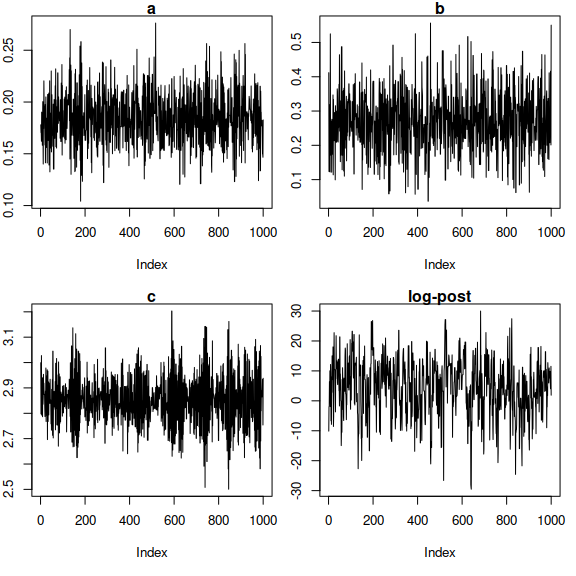Then, we can compute the $$\theta$$ parameter estimates (via posterior means) together with 95% credible intervals (via 2.5 to 97.5 percentiles of the MCMC samples) by using the summary method for a magioutput object:

summary(result, par.names = theta.names)
#>           a      b    c
#> Mean  0.185 0.2720 2.85
#> 2.5%  0.134 0.0983 2.65
#> 97.5% 0.236 0.4640 3.06

The plot method for a magioutput object can be used to visualize the inferred trajectories for each system component in $$X$$. We treat the posterior means as the inferred trajectories (black curves), and add blue shaded areas to represent the 95% credible intervals. The black points are the observed data.

plot(result, comp.names = c("V", "R"), xlab = "Time", ylab = "Level")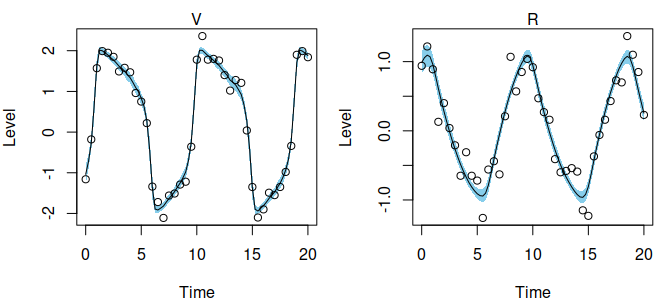The inferred trajectories appear to fit the observed data well. We can re-run MAGI at a higher discretization level to confirm that the results are stable:

yinput2 <- setDiscretization(yobs, level = 2)
result2 <- MagiSolver(yinput, fnmodel, control = list(niterHmc = 2000, nstepsHmc = 100))
summary(result2, par.names = theta.names)
#>           a      b    c
#> Mean  0.184 0.2670 2.85
#> 2.5%  0.135 0.0955 2.66
#> 97.5% 0.232 0.4380 3.06

The parameter estimates are stable between level = 1 and level = 2, indicating that discretization level is sufficient for reliable inference.

## Example with an unobserved component and asynchronous observations: Hes1 system

MAGI can handle systems with unobserved components and asynchronous observations. To illustrate, consider the three-component dynamic system $$X = (P,M,H)$$ introduced in Hirata (2002, Science) governed by the ODE $\mathbf{f}(X, {\theta}, t) = \begin{pmatrix} -aPH + bM - cP \\ -dM + \frac{e}{1 + P^2} \\ -aPH + \frac{f}{1+ P^2} - gH \end{pmatrix},$ where $$P$$ and $$M$$ are the protein and messenger ribonucleic acid (mRNA) levels in cultured cells. In experimental data, $$P$$ and $$M$$ levels exhibit oscillatory cycles approximately every 2 hours, and the $$H$$ component is a hypothesized Hes1-interacting factor the helps regulate this oscillation via a negative feedback loop. The parameters of this system are $${\theta} = (a, b, c, d, e, f, g)$$, where $$a, b$$ can be interpreted as synthesis rates; $$c,d,g$$ as decomposition rates; and $$e,f$$ as inhibition rates.

We define a function for this ODE system, that takes as input the vector of parameters $${\theta}$$ and system states $$X$$ one per column and time $$t$$, and returns an array with the values of the derivatives $$\dot{X}$$:

hes1modelODE <- function(theta, x, tvec) {
P = x[, 1]
M = x[, 2]
H = x[, 3]

PMHdt = array(0, c(nrow(x), ncol(x)))
PMHdt[, 1] = -theta * P * H + theta * M - theta * P
PMHdt[, 2] = -theta * M + theta / (1 + P^2)
PMHdt[, 3] = -theta * P * H + theta / (1 + P^2) - theta * H

PMHdt
}

Following the real experimental setup, measurements of $$P$$ and $$M$$ are taken every 15 minutes over a four hour period, but asynchronously: $$P$$ is observed at $$t = 0, 15, 30, \ldots, 240$$ minutes, while $$M$$ is observed at $$t = 7.5, 22.5, \ldots, 232.5$$ minutes, and $$H$$ is never observed.

To provide a realistic sample dataset for analysis, we simulate from this system using the parameter values studied theoretically: $$a = 0.022$$, $$b = 0.3$$, $$c = 0.031$$, $$d = 0.028$$, $$e = 0.5$$, $$f = 20$$, $$g = 0.3$$; together with initial conditions $$P(0) = 1.439$$, $$M(0) = 2.037$$, $$H(0) = 17.904$$ informed by the authors’ reported experimental data. The observation noise in the experiment is approximately 15% of both the $$P$$ and $$M$$ levels, which we treat as multiplicative noise following a log-normal distribution with known standard deviation 0.15.

For convenience, we setup a list containing these simulation values:

param.true <- list(
theta = c(0.022, 0.3, 0.031, 0.028, 0.5, 20, 0.3),
x0 = c(1.439, 2.037, 17.904),
sigma = c(0.15, 0.15, NA)
)

Note that as $$H$$ is never observed, measurement noise is not applicable to that component. Next, we use a numerical solver to construct the system trajectories implied by the parameter values and initial conditions. We can make use of the ODE solvers available in the deSolve package, by first defining a wrapper that satisfies the syntax of its ode function:

modelODE <- function(t, state, parameters) {
list(as.vector(hes1modelODE(parameters, t(state), t)))
}

We may now numerically solve the ODE trajectories over the time period of interest, from $$t=0$$ to $$4$$ hours (specified as 240 minutes):

x <- deSolve::ode(y = param.true$x0, times = seq(0, 60*4, by = 0.01), func = modelODE, parms = param.true$theta)

Next, we extract the true values of the trajectory at the time points according to the schedule of observations described, and simulate noisy measurements for $$P$$ and $$M$$. The seed 12321 is set for reproducibility of this example dataset.

set.seed(12321)
y <- as.data.frame(x[ x[, "time"] %in% seq(0, 240, by = 7.5), ])
names(y) <- c("time", "P", "M", "H")
y$P <- y$P * exp(rnorm(nrow(y), sd = param.true$sigma)) y$M <- y$M * exp(rnorm(nrow(y), sd = param.true$sigma))

For system components that are unobserved at a time point, we fill in the corresponding values with NaN, recalling that $$P$$ and $$M$$ are observed asynchronously and $$H$$ is never observed:

y$H <- NaN y$P[y$time %in% seq(7.5, 240, by = 15)] <- NaN y$M[y$time %in% seq(0, 240, by = 15)] <- NaN We plot the observed data, with the points showing the noisy measurements available for $$P$$ and $$M$$ and the solid curves showing the true system trajectories: compnames <- c("P", "M", "H") matplot(x[, "time"], x[, -1], type = "l", lty = 1, xlab = "Time (min)", ylab = "Level") matplot(y$time, y[,-1], type = "p", col = 1:(ncol(y)-1), pch = 20, add = TRUE)
legend("topright", compnames, lty = 1, col = c("black", "red", "green"))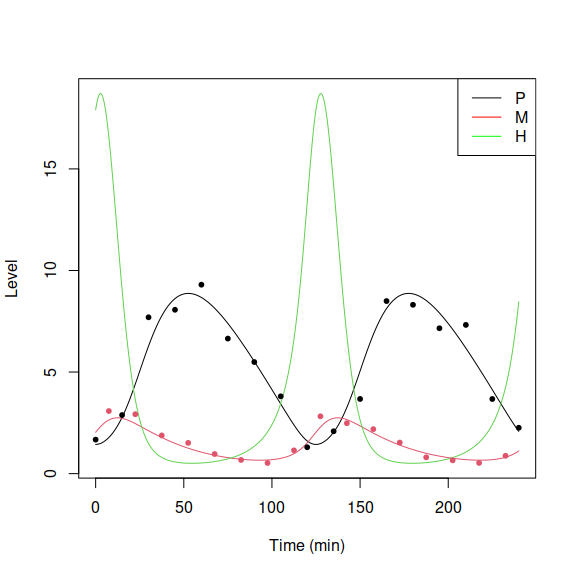Since the Hes1 observations for $$P$$ and $$M$$ are positive and subject to multiplicative error, we may apply a log-transform to the data and equations for the analysis. Apply log-transform to the data columns:

y[, names(y) != "time"] <- log(y[, names(y) != "time"])

Now the dataset y is ready for input into to infer the system trajectories and estimate the seven parameters in $${\theta}$$.

We set up the functions required for the odeModel list input to MAGI. First, we have the ODE corresponding to the log-transformed equations, namely $\mathbf{f}(X, {\theta}, t) = \begin{pmatrix} -aH' + bM'/P' - c \\ -d + \frac{e}{(1 + P'^2)M'} \\ -aP' + \frac{f}{(1+ P'^2)H'} - g \end{pmatrix}$ where $$P' = \exp(P)$$, $$M' = \exp(M)$$, and $$H' = \exp(H)$$, which we may code via the function

hes1logmodelODE <- function (theta, x, tvec) {
P = exp(x[, 1])
M = exp(x[, 2])
H = exp(x[, 3])

PMHdt <- array(0, c(nrow(x), ncol(x)))
PMHdt[, 1] = -theta * H + theta * M / P - theta
PMHdt[, 2] = -theta + theta / (1 + P^2) / M
PMHdt[, 3] = -theta * P + theta / (1 + P^2) / H - theta

PMHdt
}

Second, to facilitate HMC sampling we also supply functions for the gradients of the ODEs with respect to the system components $$X$$ and the parameters $$\theta$$. With respect to $$X$$, we have the matrix of gradients as follows,

$\frac{\partial \mathbf{f}(X, {\theta}, t)}{\partial X} = \begin{pmatrix} -b M'/P' & b M'/P' & -a H' \\ -\frac{2eP'^2}{ ( 1 + P'^2)^2 M'} & -\frac{e}{(1 + P'^2)M'} & 0 \\ -aP' - \frac{2fP'^2}{ ( 1 + P'^2)^2 H'} & 0 & -\frac{f}{(1 + P'^2)H'} \\ \end{pmatrix},$ which we specify by defining the function:

hes1logmodelDx <- function (theta, x, tvec) {
logP = x[, 1]
logM = x[, 2]
logH = x[, 3]

Dx <- array(0, c(nrow(x), ncol(x), ncol(x)))
dP = -(1 + exp(2 * logP))^(-2) * exp(2 * logP) * 2
Dx[, 1, 1] = -theta * exp(logM - logP)
Dx[, 2, 1] = theta * exp(logM - logP)
Dx[, 3, 1] = -theta * exp(logH)
Dx[, 1, 2] = theta * exp(-logM) * dP
Dx[, 2, 2] = -theta * exp(-logM) / (1 + exp(2 * logP))
Dx[, 1, 3] = -theta * exp(logP) + theta * exp(-logH) * dP
Dx[, 3, 3] = -theta * exp(-logH) / (1 + exp(2 * logP))

Dx
}

With respect to $$\theta$$, we have the matrix of gradients as follows, $\frac{\partial \mathbf{f}(X, {\theta}, t)}{\partial {\theta}} = \begin{pmatrix} -H' & M'/P' & -1 & 0 & 0 & 0 & 0 \\ 0 & 0 & 0 & -1 & \frac{1}{ ( 1 + P'^2)^2 M'} & 0 & 0 \\ -P' & 0 & 0 & 0 & 0 & \frac{1}{ ( 1 + P'^2)^2 H'} & -1 \end{pmatrix}$

which we specify by defining the function:

hes1logmodelDtheta <- function (theta, x, tvec) {
logP = x[, 1]
logM = x[, 2]
logH = x[, 3]

Dtheta <- array(0, c(nrow(x), length(theta), ncol(x)))
Dtheta[, 1, 1] = -exp(logH)
Dtheta[, 2, 1] = exp(logM - logP)
Dtheta[, 3, 1] = -1
Dtheta[, 4, 2] = -1
Dtheta[, 5, 2] = exp(-logM) / (1 + exp(2 * logP))
Dtheta[, 1, 3] = -exp(logP)
Dtheta[, 6, 3] = exp(-logH) / (1 + exp(2 * logP))
Dtheta[, 7, 3] = -1

Dtheta
}

Third, odeModel includes the upper and lower bounds on the parameters theta. Here, all of the seven parameters $$(a,b,c,d,e,f,g)$$ are non-negative, so we may set the bounds as 0 and Inf. Putting the three ODE model functions and parameter bounds together, we define the list:

hes1logmodel <- list(
fOde = hes1logmodelODE,
fOdeDx = hes1logmodelDx,
fOdeDtheta = hes1logmodelDtheta,
thetaLowerBound = rep(0, 7),
thetaUpperBound = rep(Inf, 7)
)

Additional control variables can be supplied to MagiSolver via the optional list control, which may include the following:

• sigma: a vector of noise levels (observation noise standard deviations) $$\sigma$$ for each component, at which to initialize MCMC sampling. By default, MagiSolver computes starting values for sigma via Gaussian process (GP) smoothing. If the noise levels are known, specify sigma together with useFixedSigma = TRUE.
• phi: a matrix of GP hyper-parameters for each component, with two rows for phi and phi and a column for each system component. By default, MagiSolver estimates phi via an optimization routine.
• theta: a vector of starting values for the parameters $$\theta$$, at which to initialize MCMC sampling. By default, MagiSolver uses an optimization routine to obtain starting values.
• xInit: a matrix of values for the system trajectories of the same dimension as y, at which to initialize MCMC sampling. Default is linear interpolation between the observed (non-missing) values of y and an optimization routine for entirely unobserved components of y.
• mu: a matrix of values for the mean function of the GP prior, of the same dimension as y. Default is a zero mean function.
• dotmu: a matrix of values for the derivatives of the GP prior mean function, of the same dimension as y. Default is zero.
• priorTemperature: the tempering factor by which to divide the contribution of the GP prior, to control the influence of the GP prior relative to the likelihood. Default is the total number of observations divided by the total number of discretization points.
• niterHmc: MCMC sampling from the posterior is carried out via Hamiltonian Monte Carlo (HMC). niterHmc specifies the number of HMC iterations to run. Default is 20000 HMC iterations.
• nstepsHmc: the number of leapfrog steps per HMC iteration. Default is 200.
• burninRatio: the proportion of HMC iterations to be discarded as burn-in. Default is 0.5, which discards the first half of the MCMC samples.
• stepSizeFactor: initial leapfrog step size factor for HMC. Default is 0.01, and the leapfrog step size is automatically tuned during burn-in to achieve an acceptance rate between 60-90%.
• bandSize: a band matrix approximation is used to speed up matrix operations, with default band size 20. Can be increased if MagiSolver returns an error indicating numerical instability.
• useFixedSigma: logical, set to TRUE if sigma is known. If useFixedSigma=TRUE, the known values of $$\sigma$$ must be supplied via the sigma control variable.
• verbose: logical, set to TRUE to output diagnostic and progress messages to the console.

Note that if the noise standard deviations $$\sigma$$ are known as in this example, they should be supplied via sigma in control together with useFixedSigma=TRUE. Otherwise, $$\sigma$$ will be treated as a parameter in MCMC sampling.

We can now run MAGI by calling the core function MagiSolver to sample $$X$$ and $$\theta$$ from their posterior distribution. For this run we use y as input without inserting any additional discretization points:

hes1result <- MagiSolver(y, hes1logmodel,
control = list(sigma = param.true$sigma, useFixedSigma = TRUE)) To informally assess convergence of the MCMC samples, we may examine traceplots of theta and lp: par(mfrow = c(2, 4), mar = c(5, 2, 1, 1)) theta.names <- c("a", "b", "c", "d", "e", "f", "g") for (i in 1:7) { plot(hes1result$theta[, i], main = theta.names[i], type = "l", ylab="")
}
plot(hes1result$lp, main = "log-post", type = "l", ylab = "")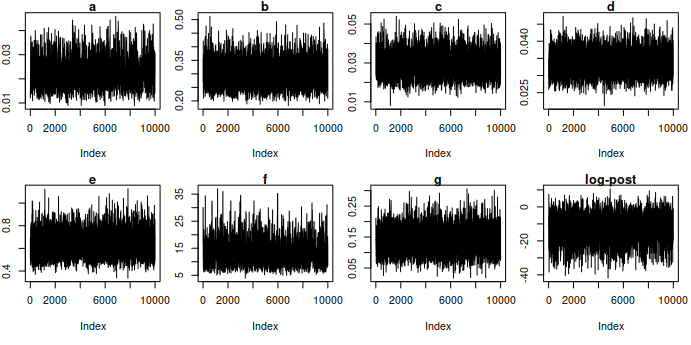Then, we can compute the $$\theta$$ parameter estimates (via posterior means) together with 95% credible intervals (via 2.5 to 97.5 percentiles of the MCMC samples): summary(hes1result, par.names = theta.names) #> a b c d e f g #> Mean 0.0218 0.301 0.0291 0.0337 0.636 13.70 0.1380 #> 2.5% 0.0121 0.225 0.0185 0.0277 0.462 6.98 0.0697 #> 97.5% 0.0350 0.402 0.0413 0.0405 0.852 25.10 0.2150 The true parameters are well-contained in the credible intervals, except for $$g$$ which governs the unobserved $$H$$ component only. We extract the sampled system trajectories $$X$$. We treat the posterior means as the inferred trajectories (green curves), and add blue shaded areas to represent the 95% credible intervals. The true trajectories are plotted in red. xLB <- exp(apply(hes1result$xsampled, c(2,3), function(x) quantile(x, 0.025)))
xMean <- exp(apply(hes1result$xsampled, c(2,3), mean)) xUB <- exp(apply(hes1result$xsampled, c(2,3), function(x) quantile(x, 0.975)))

layout(rbind(c(1, 2, 3), c(4, 4, 4)), heights = c(5, 0.5))
ylim_lower <- c(1.5, 0.5, 0)
ylim_upper <- c(10.0, 3.5, 21)
compobs <- c("17 observations", "16 observations", "unobserved")
times <- y[, "time"]

for (i in 1:3) {
plot(times, xMean[, i], type = "n", xlab = "time", ylab = compnames[i],
ylim = c(ylim_lower[i], ylim_upper[i]))
mtext(paste0(compnames[i], " (", compobs[i], ")"), cex = 1)

polygon(c(times, rev(times)), c(xUB[, i], rev(xLB[, i])),
col = "skyblue", border = NA)

lines(x[, 1], x[, 1+i], col = "red", lwd = 2)
lines(times, xMean[, i], col = "forestgreen", lwd = 2)
points(times, exp(y[, 1+i]))
}

par(mar = rep(0, 4))
plot(1, type = 'n', xaxt = 'n', yaxt = 'n', xlab = NA, ylab = NA, frame.plot = FALSE)

legend("center", c("truth", "inferred trajectory", "95% credible interval", "noisy observations"),
lty = c(1, 1, 0, 0), lwd = c(2, 2, 0, 1), col = c("red", "forestgreen", NA, "black"),
fill = c(0, 0, "skyblue", 0), text.width = c(0.02, 0.25, 0.05, 0.15), bty = "n",
border = c(0, 0, "skyblue", 0), pch = c(NA, NA, 15, 1), horiz = TRUE)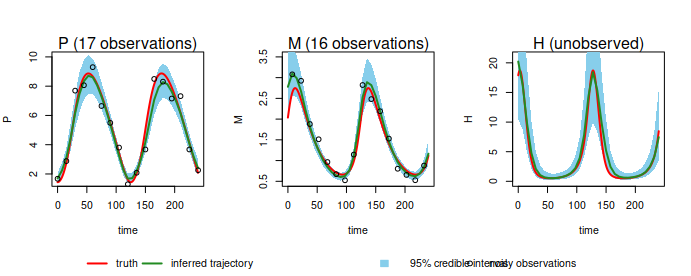The system trajectories are recovered well, including for the unobserved $$H$$ component. Similar to the FN equations, we can also verify that the results are stable if the discretization level is increased (not run here).

## Example with time-dependency in ODE: HIV model with oscillating infection rate

MAGI can accommodate systems that explicitly depend on time $$t$$. As an example, consider the three-component dynamic system $$X = (T_U,T_I,V)$$ for HIV infection simulated in Liang, Miao and Wu (2010, AOAS):

$\mathbf{f}(X, {\theta}, t) = \begin{pmatrix} \lambda - \rho T_U - \eta(t) T_U V \\ \eta(t) T_U V - \delta T_I \\ N \delta T_I - c V \end{pmatrix},$ where $$\eta(t) = 9\times 10^{-5} \times (1 - 0.9 \cos(\pi t / 1000))$$ is an oscillating infection rate over time, and the parameters to be estimated are $$\theta = (\lambda, \rho, \delta, N, c)$$. The system components $$T_U, T_I$$ refer to the concentrations of uninfected and infected cells, and $$V$$ the viral load.

We define functions for this system as in previous examples: the ODE, and gradients with respect to the system components and parameters.

hivtdmodelODE <- function(theta, x, tvec) {
TU <- x[, 1]
TI <- x[, 2]
V <- x[, 3]

lambda <- theta
rho <- theta
delta <- theta
N <- theta
c <- theta

eta <- 9e-5 * (1 - 0.9 * cos(pi * tvec / 1000))

result <- array(0, c(nrow(x), ncol(x)))
result[, 1] = lambda - rho * TU - eta * TU * V
result[, 2] = eta * TU * V - delta * TI
result[, 3] = N * delta * TI - c * V

result
}

hivtdmodelDx <- function(theta, x, tvec) {
resultDx <- array(0, c(nrow(x), ncol(x), ncol(x)))

TU <- x[, 1]
TI <- x[, 2]
V <- x[, 3]

lambda <- theta
rho <- theta
delta <- theta
N <- theta
c <- theta

eta <- 9e-5 * (1 - 0.9 * cos(pi * tvec / 1000))

resultDx[, , 1] = cbind(-rho - eta * V, 0, -eta * TU)
resultDx[, , 2] = cbind(eta * V, -delta, eta * TU)
resultDx[, , 3] = cbind(rep(0, nrow(x)), N * delta, -c)

resultDx
}

hivtdmodelDtheta <- function(theta, x, tvec) {
resultDtheta <- array(0, c(nrow(x), length(theta), ncol(x)))

TU <- x[, 1]
TI <- x[, 2]
V <- x[, 3]

delta <- theta
N <- theta

resultDtheta[, , 1] = cbind(1, -TU, 0, 0, 0)
resultDtheta[, , 2] = cbind(0, 0, -TI, 0, 0)
resultDtheta[, , 3] = cbind(0, 0, N * TI, delta * TI, -V)

resultDtheta
}

We setup a list containing some sample values for simulation that mimic the referenced paper:

param.true <- list(
theta = c(36, 0.108, 0.5, 1000, 3),
x0 = c(600, 30, 1e5),
sigma = c(sqrt(10), sqrt(10), 10),
times = seq(0, 20, 0.2)
)

and numerically solve the system trajectories over time $$t=0$$ to $$20$$ hours to mimic noisy observations every 0.1 hours with normal measurement error with SD sigma. The seed 12321 is set for reproducibility.

set.seed(12321)
modelODE <- function(tvec, state, parameters) {
list(as.vector(hivtdmodelODE(parameters, t(state), tvec)))
}

xtrue <- deSolve::ode(y = param.true$x0, times = param.true$times,
func = modelODE, parms = param.true$theta) y <- data.frame(xtrue) for(j in 1:(ncol(y) - 1)){ y[, 1+j] <- y[, 1+j] + rnorm(nrow(y), sd = param.true$sigma[j])
}

Plots of the simulated measurements:

compnames <- c("TU", "TI", "V")
par(mfrow = c(1, 3), mar = c(4, 4, 1.5, 1))
for (i in 1:3) {
plot(param.true$times, y[, i+1], xlab = "Time", ylab = complabels[i]) mtext(compnames[i]) lines(xtrue[, "time"], xtrue[, i+1], col = "red", lwd = 2) }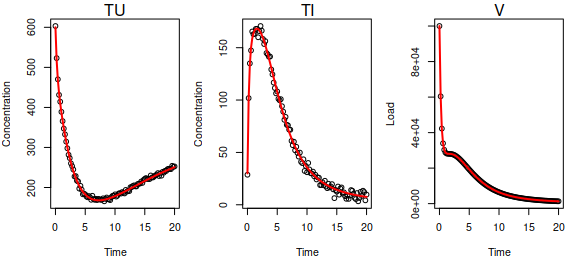We define the odeModel list input, and prepare the input y based on the observations with no additional discretization. hivtdmodel <- list( fOde = hivtdmodelODE, fOdeDx = hivtdmodelDx, fOdeDtheta = hivtdmodelDtheta, thetaLowerBound = rep(0, 5), thetaUpperBound = rep(Inf, 5) ) y_I <- setDiscretization(y, level = 1) It can be worth checking that the gradients are specified correctly. testDynamicalModel(hivtdmodelODE, hivtdmodelDx, hivtdmodelDtheta, "HIV time-dependent system", y[, 2:4], param.true$theta, y[, "time"])
#> HIV time-dependent system model, with derivatives
#> Dx and Dtheta appear to be correct
#> $testDx #>  TRUE #> #>$testDtheta
#>  TRUE

The inputs to MagiSolver are now ready. Often, MAGI’s automatic determination of hyper-parameters $$\phi$$ and initial noise levels $$\sigma$$ will be reasonable; however, this is not guaranteed, in which case we may manually override their values.

Let us explicitly call the GP smoothing procedure (gpsmoothing) included in MAGI to examine the automatic hyper-parameter setting in this case:

phiEst <- matrix(0, nrow = 2, ncol = ncol(y) - 1)
sigmaInit <- rep(0, ncol(y) - 1)
for (j in 1:(ncol(y) - 1)){
hyperparam <- gpsmoothing(y[, j+1], y[, "time"])
phiEst[, j] <- hyperparam$phi sigmaInit[j] <- hyperparam$sigma
}

colnames(phiEst) <- compnames

phiEst
#>               TU           TI            V
#> [1,] 37538.16828 11083.870501 14299.089897
#> [2,]     3.91358     2.755717     1.731937
sigmaInit
#>      3.378930     3.757803 14158.670863

Notice that initial guess of sigma for $$T_U$$ and $$T_I$$ are reasonable, while that for the $$V$$ component (green trajectory) is very large ($$>10000$$). This indicates that automatic Gaussian process smoothing is treating the sharp initial decrease of $$V$$ seen in the interval $$t=0$$ to $$t=1$$ as noise rather than signal, which would lead to undesirable results. This is accompanied by a small phi relative to the magnitude of the observations for $$V$$ and a phi that is too large (indicating that successive time points will be too highly correlated, preventing the sharp decrease from being correctly modelled), noting that phi controls the overall variance level and phi controls the bandwidth.

We can manually specify more appropriate values of phi and sigma for the $$V$$ component instead, and then supply them to MagiSolver using the optional phi and sigma control variables to override the automatic setting:

phiEst[, 3] <- c(1e7, 0.5)
sigmaInit <- 100
HIVresult <- MagiSolver(y_I, hivtdmodel,
control = list(phi = phiEst, sigma = sigmaInit))

The $$\theta$$ parameter estimates (via posterior means) together with 95% credible intervals (via 2.5 to 97.5 percentiles of the MCMC samples):

summary(HIVresult, par.names = c("lambda", "rho", "delta", "N", "c"))
#>       lambda    rho delta   N    c
#> Mean    35.9 0.1070 0.499 958 2.88
#> 2.5%    34.1 0.0982 0.494 943 2.84
#> 97.5%   37.7 0.1150 0.504 973 2.92

We extract the sampled system trajectories $$X$$. We treat the posterior means as the inferred trajectories (green curves), and the grey points are the observed data. The true trajectories (red curves) are superimposed and can be seen to be indistinguishable from the inferred trajectories.

par(mfrow = c(1, 3), mar = c(4, 3, 1.5, 1))
compnames <- c("TU", "TI", "V")
ylim_lower <- c(100, 0, 0)
ylim_upper <- c(750, 175, 1e5)

xMean <- apply(HIVresult\$xsampled, c(2, 3), mean)

for (i in 1:3) {
plot(y_I[, 1], xMean[, i], type = "n", xlab = "time", ylab = "",
ylim = c(ylim_lower[i], ylim_upper[i]))
mtext(compnames[i])
points(y_I[, 1], y_I[, i+1], col = "grey50")
lines(y_I[, 1], xMean[, i], col = "forestgreen", lwd = 4)
lines(xtrue[, "time"], xtrue[, i+1], col = "red", lwd = 1.5)
}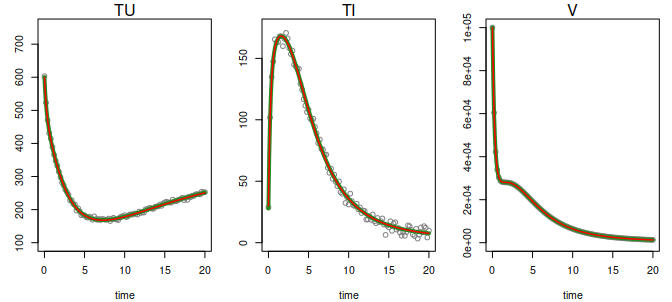par(oldpar) # reset to previous pars